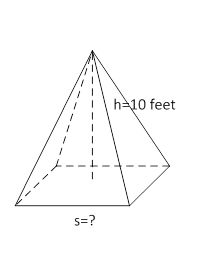### Sample Problem

The base of the following pyramid is a square. If the volume of the pyramid is 180 feet3, what is the missing length? Round the answer to the nearest hundredth.The missing length is feet.

#### Solution

Volume = 1/3 × s × s × h

So, s2 =3 × V ÷  h

=(3 × 180) ÷ 10

= 54 feet2

s == 7.35 feet